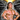# Somethinghitme

## Code, demos and ideas.

### Game Dev Movement cheatsheet with examples

November 13, 2013

#### aka My Cheat Sheet of Helpful Code

I created a blog post way back title Game Snippets I Always Forget. I put it up basically as a dumping ground for random game related bits of code I found myself looking up over.. and over. A few of them are a bit cryptic but that’s mostly due to me quickly logging in and pasting before Id forget. While now I have (most) memorized I figured I would revisit the article and add some examples that may help others out. The following are examples dealing with movement, angles, and directions.

As a disclaimer I am not claiming to be an expert in this, I’m sure there are better ways to do some of these and if there are feel free to comment and let me know.

#### Distance Check

This one is obviously very important in any sort of game development. What I find myself using it for most is quick collision checks. However its used in many cases, an example found later in this article.

``````var x = x2 - x1,
y = y2 - y1,
distance = Math.sqrt(x * x + y * y);``````

#### Move Towards an Object With Easing

This can be useful for inertia based mouse movement. This moves an object to the designated target, making it move slower the closer it gets to the target.

``````x += (targetX-x)/speed;
y += (targetY-y)/speed;``````

#### Move Towards an Object at a Constant Speed

The next bit of code can be used for any type of following behavior. The difference from the example above is that the object will move towards its target at a constant speed rather than easing its way there.

``````var tx = targetX - x,
ty = targetY - y,
dist = Math.sqrt(tx * tx + ty * ty);

velX = (tx / dist) * thrust;
velY = (ty/dist) * thrust;``````

#### Get the Angle Between Objects and Project a Point in Front of the Object

Its important to note the above example does not include the facing direction in order to get that we need to know what the angle is between both objects.

``````var x = this.x - this.targetX,
y = this.y - this.targetY,

Now that we know how to get the angle between two objects we can project a point in front of it. This can be used for positioning a gun, or for the start position of projectiles. Since realistically bullets shouldn’t necessarily come from the center of our object.

``````pointx = x + pointLength * Math.cos(radians);
pointy = y + pointLength * Math.sin(radians);``````

#### Move Towards Another Object With Proper Rotation

We can combine the two above samples and now move an object towards another with the correct facing angle. This is obviously beneficial for many reasons.

## Putting Some Information to Good Use

I assume you will find ways to use this, maybe you already have and just stumbled across this article to take a piece of code, but I wanted to throw an example or two in here just in case. The following is a quick example of how to do simple predefined pathing for tower defense enemies.

Just by using the code so far in this article you could add towers that shoot,  and implement basic collision, having most of whats needed for a very simple tower defense.

#### Move an Object Based on its Heading

The last movement example is moving based on the current heading. Meaning moving towards the angle you are facing. The angle can be a target angle, or one set by the user via the arrow keys for example. This is achieved by using the following

``````velX = Math.cos(currentAngle) * thrust;
velY = Math.sin(currentAngle) * thrust;``````

However if you want a movement style similar to asteroids you can use this instead.

``````velX += Math.cos(currentAngle) * thrust;
velY += Math.sin(currentAngle) * thrust;``````

You will most likely want to set the thrust to a lower number in the above example since you are adding it each time rather than setting it once. One tip if you are using Asteroids style movement is to apply friction or your object will go on forever. This is easily done by doing the following way.

``````velX * = 0.98;
velY * = 0.98;``````

Note you can use any value less than one to apply friction the lower the number the faster you will glide to a stop.

Regardless of the type of motion you choose you then apply the velocities to your object.

``````this.x -= this.velX;
this.y -= this.velY;``````

And here is a finished example showing the Asteroids style movement.

I hope you find this information useful.  All of the major pieces of the code needed to make many types of games are included in this post. One example where I have personally used the above information is on Retroships.com among many other projects I have worked on.

I’m considering doing another post on a few random bits of code I find myself using often, check back soon!.Written by Jason Brown Father, Veteran, lover of everything tech.